# Theano官方文档的测试和总结(1)：安装、基础语法、逻辑斯蒂回归

2020-02-01 04:53 543 查看

http://deeplearning.net/software/theano/NEWS.html

### 目录

• 2 基础语法
• 3. 实战案例：逻辑斯蒂回归
• ## 1. Theano的简介和安装

#### 1.1 Theano简介

发布版本：Theano 1.0.0 (15th of November, 2017)
Theano是一个让你去定义，优化，计算数学表达式，特别是多维数组（numpy.ndarray）的Python包。具有速度快的特点，支持GPU。Theano结合了计算机代数系统（computer algebra system ，CAS) 的特征和优化编译器的功能。

牛刀小试：

```import theano
from theano import tensor

# declare two symbolic floating-point scalars
a = tensor.dscalar()
b = tensor.dscalar()

# create a simple expression
c = a + b

# convert the expression into a callable object that takes (a,b)
# values as input and computes a value for c
f = theano.function([a,b], c)

# bind 1.5 to 'a', 2.5 to 'b', and evaluate 'c'
assert 4.0 == f(1.5, 2.5)  #检查条件，不符合就终止程序
print 4.0 == f(1.5, 2.5)    #输出Ture```

`theano.function`
可以被视为一个从纯符号图构建可调用对象的编译器接口。Theano最重要的特征之一是
`theano.function`
能够优化图，甚至能够将图的一部分或者全部编译成简单的机器指令。

#### 1.2 Theano安装

不同平台的安装方式不同，详情参考http://deeplearning.net/software/theano/install.html#install

## 2 基础语法

让我们开始一个交互式进程（使用python或者ipython), 然后倒入Theano。

`from theano import *`

Theano包的tensor模块比较常用，可以导入为T

`import theano.tensor as T`

在整个教程中，记住在每个页面的右上角都有术语表（Glossary）,索引和模块链接来帮助你。
Prerequisites： Python和NumPy

#### 2.1 蹒跚学步–代数学

两个标量（Scalars）相加

```>>> import numpy
>>> import theano.tensor as T>>> from theano import function
>>> x = T.dscalar('x')
>>> y = T.dscalar('y')
>>> z = x + y
>>> f = function([x, y], z)
```

现在，我们已经创建了函数，让我们来用它们。

```>>> f(2, 3)
array(5.0)
>>> numpy.allclose(f(16.3, 12.1), 28.4)
True```

Step 1：

`T.dscalary`
用来指定变量的类型为 “0-dimensional arrays (scalar) of doubles (d)”。 可用type查看变量类型。Theano’s inner structure参考 Graph Structures.
Step 2： z变量代表着x和y的和，可以使用pp函数打印出与z相关的计算。
Step 3： 创建一个把x,y作为输入，z作为输出的函数。也可以使用
`eval`
(串演算指令)方法，传入一个字典，返回表达式的数值。

两个矩阵（Matrices）相加

```>>> x = T.dmatrix('x')
>>> y = T.dmatrix('y')
>>> z = x + y
>>> f = function([x, y], z)
>>> f([[1, 2], [3, 4]], [[10, 20], [30, 40]])
array([[ 11.,  22.],
[ 33.,  44.]])```

dmatrix是double矩阵类型。该变量是NumPy array类型，我们也可以直接用NumPy arrays作为输入。
矩阵和标量，矩阵和向量，标量和向量的运算参考 broadcasting
变量类型，可参考 tensor creation

#### 2.2 熟悉 Theano的函数对象和操作

2.2.1 Basic Tensor Functionality

Basic Tensor Functionality
①创建浮点型矩阵：

`x = T.fmatrix()`

②变量可接受一个name。
③不同数据类型的构造函数
④各种类型的构造函数
⑤复数形式的构造函数Plural Constructors
⑥自定义tensor类型
⑦转化Python对象， 调用
`shared()`

⑧略略略

2.2.2 Logistic Function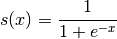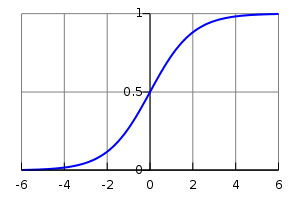可以使用elementwise来计算double型的矩阵，其意思是将每个独立的元素应用于矩阵。

```>>> import theano
>>> import theano.tensor as T>>> x = T.dmatrix('x')
>>> s = 1 / (1 + T.exp(-x))
>>> logistic = theano.function([x], s)
>>> logistic([[0, 1], [-1, -2]])
array([[ 0.5       ,  0.73105858],
[ 0.26894142,  0.11920292]])
```

logistics能够使用elementwise是因为它的所有操作都是加减乘除，是elementwise操作符。logistics函数还可以改写为如下形式：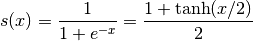再次确认这个改变：

```>>> s2 = (1 + T.tanh(x / 2)) / 2
>>> logistic2 = theano.function([x], s2)
>>> logistic2([[0, 1], [-1, -2]])
array([[ 0.5       ,  0.73105858],
[ 0.26894142,  0.11920292]])```
2.2.3 一次计算超过一个表达式

Theano支持多个输出的函数。例如，我们可以一次计算elementwise的两个矩阵的difference, absolute difference和squared difference。

```>>> a, b = T.dmatrices('a', 'b')
>>> diff = a - b
>>> abs_diff = abs(diff)
>>> diff_squared = diff**2
>>> f = theano.function([a, b], [diff, abs_diff, diff_squared])```

注意：dmatrice可以产生很多的输出。它是分配符号变量（allocating symbolic variables）的简写。
当我们使用函数f, 它会返回三个变量（打印被重新格式化为可读的形式）。

```>>> f([[1, 1], [1, 1]], [[0, 1], [2, 3]])
[array([[ 1.,  0.],
[-1., -2.]]), array([[ 1.,  0.],
[ 1.,  2.]]), array([[ 1.,  0.],
[ 1.,  4.]])]   #三个array分别代表着三个输出。```
2.2.4 设置带默认值的申明argument
```f = function([x, In(y, value=1)], z)    #使用In类型来更加详细地指定你的函数的参数的属性。
f = function([x, In(y, value=1), In(w, value=2, name='w_by_name')], z)```
2.2.5 使用共享变量

应用场景：使函数具有内部状态。例如，我们想要创建一个累加器（accumulator）： 初始状态为0，每调用一次函数，函数申明的状态值都增加。
首先让我们定义一个累加器（accumulator）函数。它增加它的参数到内部状态，然后返回老的状态值。

```>>> from theano import shared
>>> state = shared(0)
>>> inc = T.iscalar('inc')
>>> accumulator = function([inc], state, updates=[(state, state+inc)])```

上述代码中，

`shared`
函数创建了一个shared variables。这些混合的符号和非符号变量的值可以被多个函数共享。共享变量能够被用于如
`dmatrices(...)`
返回的对象所示的符号表达式，但是这些符号有内部值，该内布值定义了使用该共享变量的所有函数的符号变量的值。
共享变量的相关操作：
`.get_value()`
`.set_value()`
分别用于获取和修改共享变量的值。
要点归纳：
①使用
`function`
`updates`
参数来更新函数的参数。使用形式为 (shared-variable, new expression)，或者键值对。
②为什么updates机制存在？ 语法方便；在原地算法（in-place algorithms，例如低阶矩阵、快速排序）中更快；GPU的良好表现。
③使用共享变量定义了表达式，但是不想使用它的值怎么办？ 使用function的givens参数来替代特定函数图中的特定节点。实际上，givens是一种允许你使用一个不同的表达式（该表达式计算出一个相同性状和数据类型的张量）替换公式的任何部分的机制。

注意：
Theano的共享变量的broadcast pattern在每一维上默认是False。共享变量的大小随时间变化，因此我们不能够使用shape来查找broadcastable pattern。如果你想要一个不一样的模式，可以传递一个参数：

`theano.shared(..., broadcastable=(True, False))`

小贴士：什么是broadcast pattern？
参考：Common Data Integration Patterns -The Broadcast Pattern
说明：当考虑Dynamics 365（微软发布的企业资源规划（ERP）和客户关系管理（CRM）应用程序生产线）多种多样的 整合需要时，解决这些问题的公共设计模式出现了。设计模式，在软件工程中是解决特定类型需求的最符合逻辑顺序的步骤，是从实际应用案例中建立的。最常见的数据整合方式有5种。
broadcast pattern是事务性的（transactional），意味着如果数据迁移（一个事务“transaction”）成功，数据被提交（即持久化）到目的地。如果事务失败，数据迁移失败或者回滚。这种类型的同步还优化了处理记录的速度，以便随着时间的推移，多个系统之间的数据是最新的。因此，广播集成必须具有高可用性和可靠性，以避免在传输过程中丢失关键数据。这就是企业服务总线（Enterprise Service Bus）至关重要的地方。

2.2.6 复制函数

Theano的函数可以被复制，针对于创建相似但是具有不同的共享变量的函数很有用。
通过function对象的

`copy()`
方法来实现。

2.2.7 使用随机数

将随机数放到Theano中计算就是把随机数放到你的图中。
Theano将会给每个变量分配一个NumPy RandomStream object（一个随机数生成器），需要时可从中提取。我们把这种随机数序列称为随机流。随机流位于它们的核心共享变量中，因此对共享变量的观察也适用于此。Theano的随机对象是在RandomStreams中定义和实现的，在较低的级别上是在RandomStreamsBase中。

Brief Example

```from theano.tensor.shared_randomstreams import RandomStreams
from theano import function
srng = RandomStreams(seed=234)
rv_u = srng.uniform((2,2))    #代表一个取自均匀分布的2x2矩阵的随机输入流，由RandomStreams的raw_random实现
rv_n = srng.normal((2,2))     #代表一个取自正态分布的2x2矩阵的随机输入流，由RandomStreams的raw_random实现
f = function([], rv_u)        #调用f函数时，可返回不同的结果；而调用g函数时，返回结果相同。
g = function([], rv_n, no_default_updates=True)    #Not updating rv_n.rng，返回结果不影响随机数产生器的状态
nearly_zeros = function([], rv_u + rv_u - 2 * rv_u)  #随机变量在单个函数中最多调用一次，nearly_zeros恒为0```

Seeding Streams
随机变量的种子（seed）可能是单独的，也可以是集体的。
单独的：用

`.rng.set_value()`
，通过设置
`.rng`
属性，来给一个随机变量设置种子。
集体的：通过RandomStream的对象的seed方法给所有的随机变量分配种子。

Sharing Streams Between Functions
与通常的共享变量一样，用于随机变量的随机数生成器在函数之间是通用的。

Copying Random State Between Theano Graphs
在某些使用情况下，一个用户可能想要转移跟给定的theano graph（如g1, 编译函数f1）相关的所有随机数产生器的状态到第二个graph(如g2， 编译函数f2)。

## 3. 实战案例：逻辑斯蒂回归

```import numpy
import theano
import theano.tensor as Trng = numpy.random

N = 400                                   # training sample size
feats = 784                               # number of input variables

# generate a dataset: D = (input_values, target_class)
# numpy.random.randn(d0, d1, ..., dn) 返回一个标准的正态分布，数组的性状为(d0, d1, ..., dn)
# numpy.random.randint(low, high=None, size=None, dtype='l')返回从low（含）到high（不含）的随机整数。
D = (rng.randn(N, feats), rng.randint(size=N, low=0, high=2))
training_steps = 10000

# Declare Theano symbolic variables
x = T.dmatrix("x")  #返回2维的float64浮点数数组
y = T.dvector("y")  #返回1维的float64浮点数向量

# initialize the weight vector w randomly
#
# this and the following bias variable b
# are shared so they keep their values
# between training iterations (updates)
w = theano.shared(rng.randn(feats), name="w") #生成一个1维的含有784个值的数组

# initialize the bias term
b = theano.shared(0., name="b")

print("Initial model:")
print(w.get_value())
print(b.get_value())

# Construct Theano expression graph
# theano.tensor.dot(X, Y) 对于2维数组等同于矩阵操作，返回X和Y的乘积
p_1 = 1 / (1 + T.exp(-T.dot(x, w) - b))   # Probability that target = 1
prediction = p_1 > 0.5                    # The prediction thresholded
xent = -y * T.log(p_1) - (1-y) * T.log(1-p_1) # Cross-entropy loss function
cost = xent.mean() + 0.01 * (w ** 2).sum()# The cost to minimize
gw, gb = T.grad(cost, [w, b])             # Compute the gradient of the cost
# w.r.t weight vector w and
# bias term b
# (we shall return to this in a
# following section of this tutorial)

# Compile
train = theano.function(
inputs=[x,y],
outputs=[prediction, xent],
updates=((w, w - 0.1 * gw), (b, b - 0.1 * gb)))
predict = theano.function(inputs=[x], outputs=prediction)

# Train
for i in range(training_steps):
pred, err = train(D, D)

print("Final model:")
print(w.get_value())
print(b.get_value())
print("target values for D:")
print(D)
print("prediction on D:")
print(predict(D))
```

运行结果如下：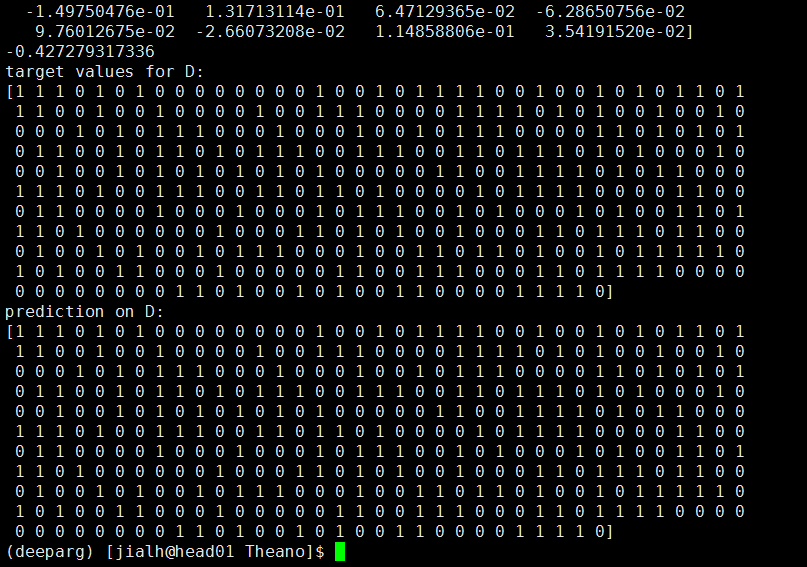• 点赞
• 收藏
• 分享
• 文章举报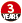LonghaoJia1997 发布了2 篇原创文章 · 获赞 1 · 访问量 1172 私信 关注
内容来自用户分享和网络整理，不保证内容的准确性，如有侵权内容，可联系管理员处理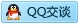标签：
相关文章推荐
新的分享
章节导航